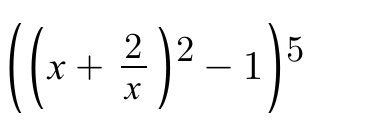# Doubt from binomial theorem

\left(x^2+\frac{4}{x^2}+3 \right) ^5=(t+3)^5
Expand in terms of t and then expand t in terms of x
Also there is another method so called multinomial theorem.
I don't know much about that. @Achyut_2020

1 Like
2 Likes

Answer is b 1103 Likes

Yes @Manu_2020

2 Likes